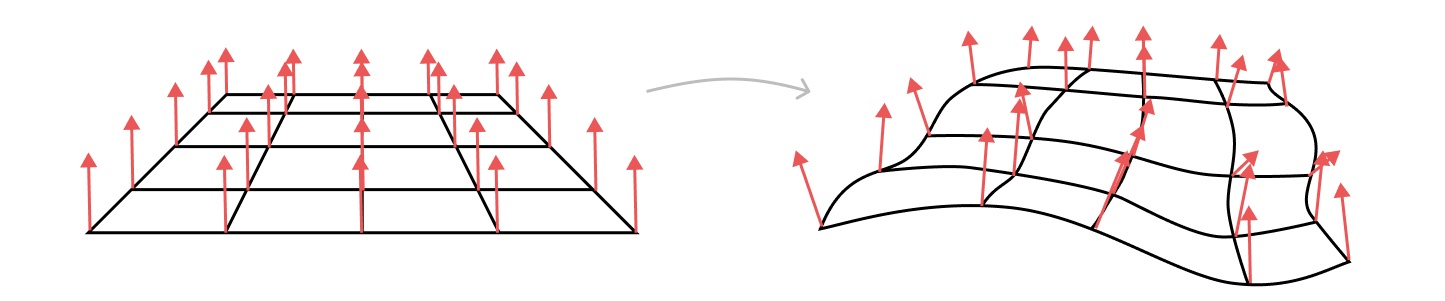# GLSL Autodiff

August 13, 2021

Tired of doing math to get normals in your vertex shader? Same. Use this library to write your function once and generate derivatives automatically!

## Why?

Sometimes, I want to displace mesh vertices in a vertex shader. After doing this, the normals of a surface should change:After distorting the vertices in a mesh, the original normals may no longer be correct

However, per-vertex normals don't automatically update! Manual updating of vertex normals requires you to take the derivative of your displacement function. This gets pretty involved and error-prone, so I wrote this library to automate the process. I wrote a long writeup on the math involved if you're interested in the internals of how it works.

Instead of writing GLSL code to first compute an offset and then compute a new normal induced by that offset, GLSL Autodiff lets you write just the displacement function using a Javscript-based API, and it will generate GLSL code for you for both the displacement and the normal!

``````import { gen } from `@davepagurek/glsl-autodiff'

const vert = `
void main(void) {
vec4 objSpacePosition = vec4(aPosition, 1.0);

float x = objSpacePosition.x;
float y = objSpacePosition.y;
// Compute an offset using the uniforms and attributes in the shader
const speedX = 1.5
const speedY = 2.8

for (let i = 0; i < 3; i++) {
time.mult(0.002),
)
))
}
offset = offset.mult(0.1)

// Generate GLSL code for the offset
offset.output('z')

// Generate GLSL code for the derivative
offset.outputDeriv('dzdx', x)
offset.outputDeriv('dzdy', y)
})}

// Use the generated displacement
objSpacePosition.z = z;

// Calculate the normal from the auto-generated derivatives
vec3 slopeX = vec3(1.0, 0.0, dzdx);
vec3 slopeY = vec3(0.0, 1.0, dzdy);
vNormal = uNormalMatrix * normalize(cross(slopeX, slopeY));

vec4 worldSpacePosition = uModelViewMatrix * objSpacePosition;
gl_Position = uProjectionMatrix * worldSpacePosition;
}
`
``````

## Using the library

Install the library via yarn with:

``````yarn add @davepagurek/autodiff
``````

Then, you can import it in your Javascript or Typescript code:

``````import { gen } from `@davepagurek/glsl-autodiff'
``````

Full API usage is available on GitHub.

## Example Output

I've used this library a few times now to bend 3D models and still have working normals: